# What are fractions?#### All in One Place

Everything you need for better grades in university, high school and elementary.#### Learn with Ease

Made in Canada with help for all provincial curriculums, so you can study in confidence.#### Instant and Unlimited Help

0/4
##### Intros
###### Lessons
1. Introduction to Fractions:
2. What are fractions?
3. How to write fractions using numbers and shapes
4. How to write fractions to represent groups of objects
5. How to show fractions on number lines
0/25
##### Examples
###### Lessons
1. Equal Parts of a Whole
How many equal parts are there? Each part is how much of a whole?
1.2.3.2. Counting Shaded Parts of a Whole
What fraction is shown by the shaded part(s)?
1.2.3.3. Shading in Parts of a Whole
Represent the fraction by shading in parts of the shape
1. $\frac{5}{6}$ of this hexagon:2. $\frac{4}{7}$ of this hexptagon:3. $\frac{1}{3}$ of this triangle:4. Fractions - Word Problem 1
There were 10 kittens at the pet store. By the end of the day, 3 of them had been adopted.
1. What fraction of kittens were adopted?
2. What fraction of kittens were NOT adopted?
5. Fractions - Word Problem 2
Stella has a butterfly collection at home. She has 5 monarch butterflies, 2 swallowtail butterflies, 8 white cabbage butterflies, and 1 zebra butterfly.
1. What fraction of her collection is swallowtail butterflies?
2. What fraction of her collection is NOT zebra butterflies?
3. What fraction of her collection does the monarch and white cabbage butterflies make up together?
6. Guessing the fraction by creating equal parts
What fraction of the picture is shown by the shaded area? Try to split each into equal parts first.
1.2.3.4.7. Writing the fraction represented by the number line
Write the fraction shown by the dot on the number line.
1.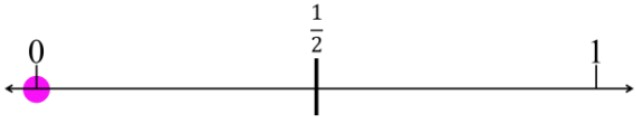2.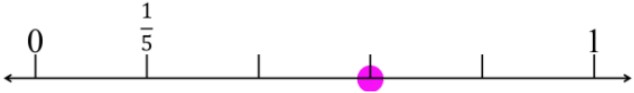3.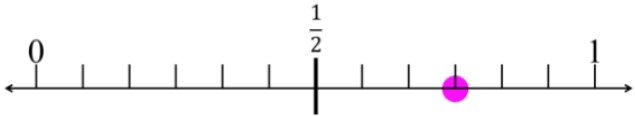8. Fractions for sets of objects
Describe the sets of objects with fractions by filling in the blanks.
1. There are ___ balls in total
___ are yellow out of ___ balls
What fraction of the balls are yellow? ___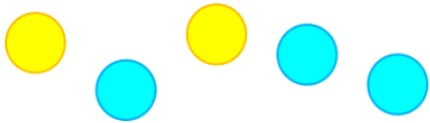2. There are ___ cubes
___ are green out of a total of ___ cubes
What fraction of the cubes are green? ___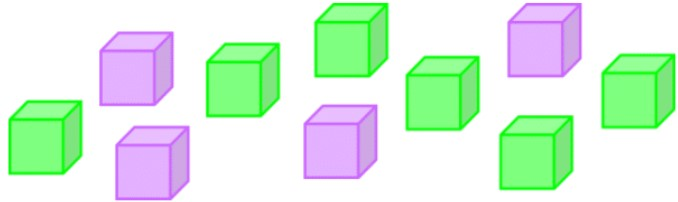3. If one-fourth of the light bulbs are broken:

There are ___ light bulbs in total
___ lightbulb(s) work and ___ lightbulb(s) do not work
What fraction of lightbulbs are not broken? ___4. Three slices of pizza have been eaten but five slices of pizza are left over:

There were ___ slices of pizza in total
___ slices have been eaten and ___ slices have not been eaten yet
What fraction of pizza slices have been eaten? ___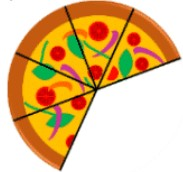0%
##### Practice
###### Topic Notes

In this lesson, we will learn:

• How to understand and write fractions using shape models
• How to understand and write fractions using groups of objects
• How to show fractions on number lines

Notes:

• Fractions are parts of a whole!
• Fractions represent smaller pieces of a total (whole) thing
• Whole shapes are divided into equal parts
• Whole groups are divided into equal smaller groups (number of items)

• If you have $n$ number of equal parts of a shape, then each part is worth $\frac{1}{n}$ of a whole.
• Ex.8 equal parts; each part is $\frac{1}{8}$ of a whole

• When writing a fraction with numbers, there are two parts:
• The top (the “numerator”) represents how many parts you have
• The bottom (the “denominator”) represents how many total parts the whole is divided into
• Ex.• Fractions can also represent groups of objects.
• Ex. what fraction of these jellybeans are green?

•• If you were to count:
• all the jellybeans it would be: $\frac{12}{12}$ jellybeans
• the jellybeans that were NOT green: $\frac{12 total -3 green}{12} = \frac{9}{12}$ not green
• the jellybeans that ARE green and NOT green: $\frac{3}{12} + \frac{9}{12} = \frac{12}{12}$ jellybeans
• if you add all the different parts of the whole, you will end up with the whole (when the top is equal to the bottom)